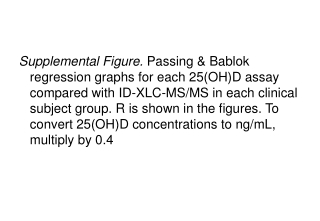DownloadDownload PresentationHealthy individuals

Healthy individuals

Télécharger la présentationHealthy individuals

- - - - - - - - - - - - - - - - - - - - - - - - - - - E N D - - - - - - - - - - - - - - - - - - - - - - - - - - -
Presentation Transcript

1. Supplemental Figure. Passing & Bablok regression graphs for each 25(OH)D assay compared with ID-XLC-MS/MS in each clinical subject group. R is shown in the figures. To convert 25(OH)D concentrations to ng/mL, multiply by 0.4

2. Healthy individuals R = 0.93 R = 0.94 R = 0.97 R = 0.92 R = 0.97 R = 0.94

3. Pregnant women R = 0.92 R = 0.88 R = 0.91 R = 0.92 R = 0.92 R = 0.96

4. Hemodialysis patients R = 0.93 R = 0.86 R = 0.92 R = 0.93 R = 0.97 R = 0.84

5. Intensive care patients R = 0.81 R = 0.83 R = 0.94 Intensive care patients not determined with iSYS R = 0.91 R = 0.82